The Mechanical Drawing Exercises ClipArt gallery provides 32 examples of practice exercises for persons studying mechanical drawing.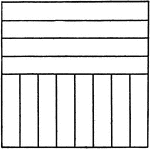### Drawing Exercise for T-square, Triangle, and Scale

"Through the center of the space draw a horizontal and a vertical line, measuring on these lines as…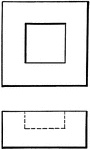### Drawing Lines 1

Dotted lines can indicate a relationship among figures, such as one inside another.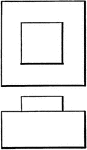### Drawing Lines 2

Shading of the right and lower sides gives dimension to drawings.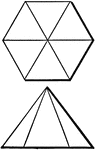### Drawing Lines 3

Top and side views of a hexagonal pyramid shaded with dimension lines.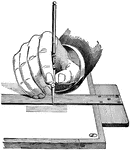### Hand Drawing

A hand demonstrating inking parallel lines using a t-square and a drafting board.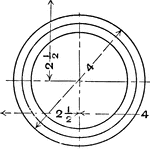### Mechanical Drawing Exercise Circle with Wavy Curved Lines Inside

A mechanical drawing exercise of a circle with curved wavy lines. The image is drawn by dividing horizontal…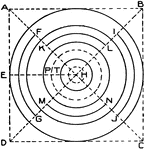### Circle Exercise

Circle pattern exercise: Draw diagonals A C and D B, and with the T-square draw the line E H. Now mark…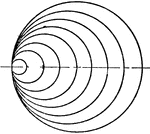### Curved Line with Circle Mechanical Drawing Exercise

A mechanical drawing exercise of drawing a curved line with circle in the middle. The image is drawn…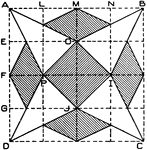### Diamond Exercise

Diamond pattern exercise: Divide A D and A B into 4 equal parts, then draw horizontal lines through…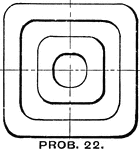### Drawing Exercise Eight Concentric Circles using Ink

A drawing exercise for drawing eight concentric circles by dividing the paper in quarters as shown.…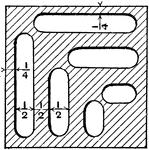### Dotted Lined Eight Concentric Circles using Ink and Pencil Drawing Exercise

Mechanical drawing exercise of drawing dotted lined concentric circles. The circles are first drawn…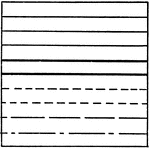### Horizontal Solid and Dashed Line Drawing Exercise

An exercise for mechanical drawing to construct a series of horizontal lines. The solid lines are drawn…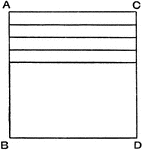### Horizontal Solid Line Drawing Exercise

An exercise problem drawing a series of horizontal lines using a straight edge or triangle without using…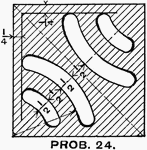### Drawing Exercise of Inscribed Circle Pattern Tangent to Left Side

An inscribed circle pattern exercise for mechanical drawing. The pattern is drawn by dividing the horizontal…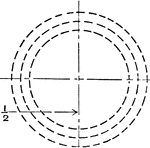### Inscribed Circle with Smaller Circles at Tangent Points Both Sides of the Large Circle Mechanical Drawing Exercise

Mechanical drawing exercise for circle with two tangent points on both sides of the circle. The paper…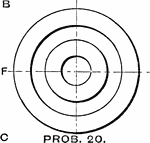### Mechanical Drawing Exercise Line Shading Plates with Holes in Ink

A practice exercise for mechanical drawing to line shade the plate with elliptic holes. The plate is…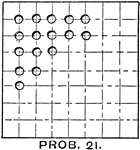### Mechanical Drawing Exercise Line Shading Plates with Joining Arcs in Ink

"This is a practice in the joining of shaded arcs, the smaller of which should be drawn with the bow—Pen,…### Mechanical Drawing Exercise Shading Sides of Inscribed Circle with Ink

A mechanical drawing practice problem of shading the sides of the inscribed circles. The darker area…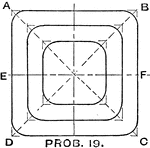### Mechanical Drawing Exercise Shading Sides of Inscribed Squares and Circle with Ink

Mechanical drawing exercise of shading squares and circle in ink. The squares are first drawn with the…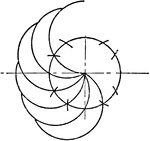### Mechanical Drawing Exercise Shading Sides of Small Circles with Bow Pen

An exercise to shade small circles with bow pen for mechanical drawing. The paper is first divided into…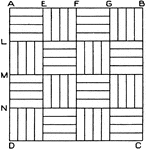### Square Exercise

Square pattern exercise: Divide A D and A B into 4 equal parts and draw horizontal and vertical lines.…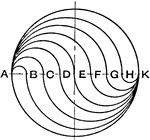### Mechanical Drawing Exercise Three Inscribed Squares with Rounded Corners using Triangle and Bow

"With a 45 degree triangle draw lines AC and BD through the center and construct three squares. Set…### Weave Exercise

Weave pattern exercise: Divide A D and A B into 8 equal parts, and through the points O, P, Q, H, I,…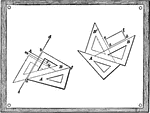### Mechanical Drawing Exercise

Drawing perpendicular lines using a drawing board and pairs of triangles.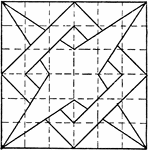### Constructing 4 Point Star Overlapping Box Pattern using T Square and Triangle

A problem exercise to construct a star overlapping square using a triangle and T square. The image is…### Constructing Star and Box Overlap Pattern using T Square and Triangle

The construction of a star with square overlapping each other using a triangle and T square. This image…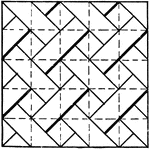### Drawing Exercise for Drawing Diagonal Line Pattern using T Square and Triangle

A diagonal line pattern drawing exercise in for T square and triangle. The image is constructed by dividing…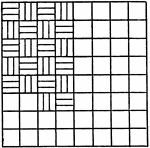### Inscribed Alternating Horizontal and Vertical Lines Repeating Patterns

An exercise of creating a series of repeating and alternating vertical and horizontal lines in an equally…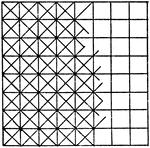### Squares Diagonal Lines Repeating Patterns

A problem exercise using a t square and 45 degree triangle to divide the paper into half inch squares.…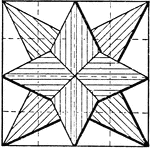### Constructing Star Using Pentagons

An illustration of drawing an eight point star using a series of pentagons to create a star. The paper…